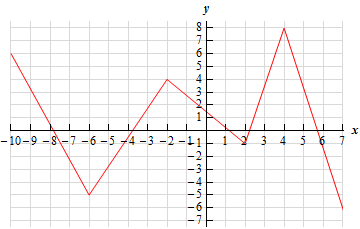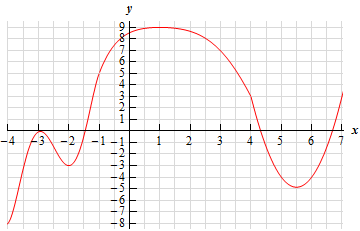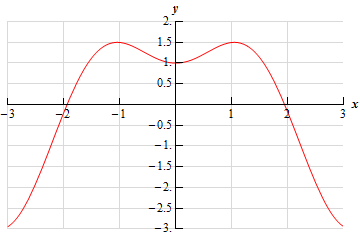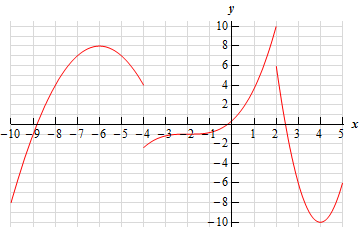Paul's Online Notes
Home / Calculus I / Applications of Derivatives / Minimum and Maximum Values
Show Mobile Notice Show All Notes Hide All Notes
Mobile Notice
You appear to be on a device with a "narrow" screen width (i.e. you are probably on a mobile phone). Due to the nature of the mathematics on this site it is best views in landscape mode. If your device is not in landscape mode many of the equations will run off the side of your device (should be able to scroll to see them) and some of the menu items will be cut off due to the narrow screen width.
Assignment Problems Notice
Please do not email me to get solutions and/or answers to these problems. I will not give them out under any circumstances nor will I respond to any requests to do so. The intent of these problems is for instructors to use them for assignments and having solutions/answers easily available defeats that purpose.

If you are looking for some problems with solutions you can find some by clicking on the "Practice Problems" link above.

### Section 4.3 : Minimum and Maximum Values

1. Below is the graph of some function, $$f\left( x \right)$$. Identify all of the relative extrema and absolute extrema of the function.2. Below is the graph of some function, $$f\left( x \right)$$. Identify all of the relative extrema and absolute extrema of the function.3. Below is the graph of some function, $$f\left( x \right)$$. Identify all of the relative extrema and absolute extrema of the function.4. Below is the graph of some function, $$f\left( x \right)$$. Identify all of the relative extrema and absolute extrema of the function.5. Sketch the graph of $$f\left( x \right) = 3 - {\frac{1}{2}}x$$ and identify all the relative extrema and absolute extrema of the function on each of the following intervals.
1. $$\left( { - \infty ,\infty } \right)$$
2. $$\left[ { - 3,2} \right]$$
3. $$\left[ { - 4,1} \right)$$
4. $$\left( {0,5} \right)$$
6. Sketch the graph of $$g\left( x \right) = {\left( {x - 2} \right)^2} + 1$$ and identify all the relative extrema and absolute extrema of the function on each of the following intervals.
1. $$\left( { - \infty ,\infty } \right)$$
2. $$\left[ {0,3} \right]$$
3. $$\left[ { - 1,5} \right]$$
4. $$\left[ { - 1,1} \right]$$
5. $$\left[ {1,3} \right)$$
6. $$\left( {2,4} \right)$$
7. Sketch the graph of $$h\left( x \right) = {{\bf{e}}^{3 - x}}$$ and identify all the relative extrema and absolute extrema of the function on each of the following intervals.
1. $$\left( { - \infty ,\infty } \right)$$
2. $$\left[ { - 1,3} \right]$$
3. $$\left[ { - 6, - 1} \right]$$
4. $$\left( {1,4} \right]$$
8. Sketch the graph of $$h\left( x \right) = \cos \left( x \right) + 2$$ and identify all the relative extrema and absolute extrema of the function on each of the following intervals. Do, all work for this problem in radians.
1. $$\left( { - \infty ,\infty } \right)$$
2. $$\left[ - \frac{\pi }{3},\frac{\pi }{4}} \right$$
3. $$\left[ - \frac{\pi }{2},2\pi } \right$$
4. $$\left[ \frac{1}{2},1} \right$$
9. Sketch the graph of a function on the interval $$\left[ {3,9} \right]$$ that has an absolute maximum at $$x = 5$$ and an absolute minimum at $$x = 4$$.
10. Sketch the graph of a function on the interval $$\left[ {0,10} \right]$$ that has an absolute minimum at $$x = 5$$ and an absolute maximums at $$x = 0$$ and $$x = 10$$.
11. Sketch the graph of a function on the interval $$\left( { - \infty ,\infty } \right)$$ that has a relative minimum at $$x = - 7$$, a relative maximum at $$x = 2$$ and no absolute extrema.
12. Sketch the graph of a function that meets the following conditions :
1. Has at least one absolute maximum.
2. Has one relative minimum.
3. Has no absolute minimum.
13. Sketch the graph of a function that meets the following conditions :
1. Graphed on the interval $$\left[ {2,9} \right]$$.
2. Has a discontinuity at some point interior to the interval.
3. Has an absolute maximum at the discontinuity in part (b).
4. Has an absolute minimum at the discontinuity in part (b).
14. Sketch the graph of a function that meets the following conditions :
1. Graphed on the interval $$\left[ { - 4,10} \right]$$.
2. Has no relative extrema.
3. Has an absolute maximum at one end point.
4. Has an absolute minimum at the other end point.
15. Sketch the graph of a function that meets the following conditions :
1. Has a discontinuity at some point.
2. Has an absolute maximum and an absolute minimum.
3. Neither absolute extrema occurs at the discontinuity.